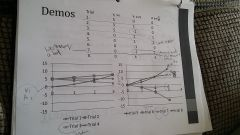• Shuffle
Toggle On
Toggle Off
• Alphabetize
Toggle On
Toggle Off
• Front First
Toggle On
Toggle Off
• Both Sides
Toggle On
Toggle Off
Toggle On
Toggle Off
Front

### How to study your flashcards.

Right/Left arrow keys: Navigate between flashcards.right arrow keyleft arrow key

Up/Down arrow keys: Flip the card between the front and back.down keyup key

H key: Show hint (3rd side).h key

A key: Read text to speech.a keyPlay buttonPlay buttonProgress

1/30

Click to flip

### 30 Cards in this Set

• Front
• Back
 Velocity= displacement/timeAverage Velocity: (displacement for trip)/time Average Velocity= 45m/3s= 15m/s or1/2(vi+vf)= 1/2(30m/s+0)= 15m/s Acceleration rate of change of velocity or directionA= (vf-vi)/(tf-ti)change in velocity/change in time If you are driving on a circular track at a constant speed, is acceleration zero? No, because constantly changing direction, so that change is an acceleration Xi = inchesvi = velocity in/sa = accecleration in/s2Newtons first law object at rest stays are restin motion stays in motion Newton's Second Lawunits for force?Weight? Force is proportional to accelerationForce = Mass x Acceleration1 Newton= force to move 1kg by 1m/s2Weight is in Newtons= kgx 9.8m/s2 Tennis Ball and bowling ball are dropped.Does one have a greater force, neglecting air resistance? Although the acceleration is the same, the bowling ball requires more force (Newtons) to accelerate down because F= Mass kg x Acceleration in m/s2 What is constant acceleration?If a ball is thrown straight up, is it still accelerating when it stops? meaning constant force appliedF= Mass kg x Acceleration in m/s2Yes, still accelerating Can object move up or down with decreasing velocity?Can speed be negative? YesNo, speed is positive, so if showing a speed curve on a ball thrown up, it slows to zero and speeds back up. Is a ball resting on a table accelerating? Yes, table is providing an opposite force. Newtons Third Law For every action, there is an equal and opposite reaction. Example of a scalar?How do we relate these to vectors? TemperatureCan only multiply and divide, so act as a coefficient-1X 3N= -3N2x2N= 4 N Work definitionFormulaunits? Force applied over distanceW= Force(N) x Displacement (M)= so, Newtonxmeters, or W= MxAxD= (kgxm)/s2 x (m)Joules =Kgxm2/s2only force in direction of displacement counts towards work Kinetic Energy definitionFormula?If you roll a 1kg ball on the floor and exert 6N over 0.2M, What is the kinetic energy? Energy associated with the motion of an objectKE= Fxd for initial calculation= NxM=JKE= (1/2)(mass)(v2)Work is the kinetic energy, only use other formula to solve for final speed. so KE= W= (kg already factored)6Nx0.2M= 1.2 J 0.1 kg ball6N forceover 2MWhat is the KE?What is the final velocity? KE = W= MAd= Fd= 6Nx0.2M= 1.2JKE= 1/2mv2, so1.2J= (1/2)(0.1kg)(v2)=(1.2J)/(1/2)(0.1kg)= v2=4.9 m/s How do you calculate final velocity? Plug total Joules in as KE for KE=1/2 mv2and solve for V 4kg ball rolling on flat surface at 2 m/s6N force is applied over 0.5m in opposite directioninitial kinetic energy?Work by force?Final Kinetic energy?Final Velocity? Initial KE= (1/2)4kgx (2m/s)2= 8Jwork by forced= 6Nx0.5M= 3JFinal KE = 8J-3J= 5JVf? 5J= (1/2) 4kg (v2)= (5J)/(1/2)4kg= v2= 1.6 m/s Potential Energy Same as calculating Work =FxDwhere distance is height. PE= mgh ormass x acceleration x height Calculate the potential energy of 2kg box on shelf 1.5m high PE= mghPE= (2kg)(9.8m/s2)(1.5m)=29J Relate the Law of conservation of energy to Potential Energy Energy cannot be created or destroyed, therefore 29J of Potential Energy can be converted to 29J of Kinetic Energy What if 10J of energy is used to push a box along a surface? Does it have 10J PE? No, 10J was lost in the form of heat energy or friction 4kg box 1.5m off the ground. What is its speed when it hits the ground? PE= mghso - (4kg)(9.8m/s2)(1.5m)= 59JThen KE=1/2mv259J= (1/2) 4kg v2=5.4 m/s Skier on top of 30 degree hill, what is speed at bottom?55kg 100m high PE= mgh55kgx100mx9.8= 53900J= PE which is converted to KEKE= 1/2mv253900J=1/2 (55kg) v2Speed= 44.3 m/sEasier way for velocity with gravity=V= root(2gh) or V=root(2x9.8x100m)= 44.3 m/s What if hill is bumpy or goes up or down same speed because energy is conserved 55kg skier100m hillHow much energy is lost to "other forces" if final velocity is 10m/s? PE= mgh= 55x9.8x100m= 53,900JKE= 1/2mv2 = 1/2 (55kg) (10m/s2)=2750JSo for loss, take PE at top- KE at Bottom=51,150J lost to other forces 55kg skier already traveling at 10m/sat top of 100m hill What is their speed at bottom assuming no other factors are at play? Total energy at top is PE + KESo PE = mgh= 55x9.8x100= 53900KE= 1/2 55kg x (10)2 = 2750Total energy at top= 56,650For speed KE =mv2or 56650= 1/2(55kg)x (v)2= 45.4 m/s not much different PowerUnitFormula? The rate that work is done1J/per second= 1 WattJoules/secondPower=work/timePower = (mgh)/time average power output hiker 55kg who climbs 1300m in 2h Power= mgh/t55x9.8x1300/(2h)need seconds55x9.8x1300/ 7200=97.3 Watts or Joules/sec Momentumsign?definition Momentum is vector quantitiysignified by little p or pForce = rate of change of momentum momentum p Momentum greater, 100kg man at 2m/sor 2000kg car at 1 m/s? p= Mass x VelocityMan p = 100kg x 2 m/s= 200Car p = 2000kg x 1 m/s = 2000car is greater